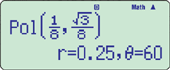Out of Stock

## CASIO fx-350ES PLUS

6.600 BD

Out of stock

SKU: 4971850090663 Category:

## Description

### New functions

• Prime factorizations
• Random integers

### Standard functions

• Fraction calculations
• Combination and permutation
• Statistics (List-based STAT data editor, standard deviation, regression analysis)
• 9 variables
• Table function
• Comes with new slide-on hard case
• #### Coordinate transformation• #### Power calculation#### Statistics

•••#### Trigonometry

•#### Table function

••••••## Specifications

• Non Programmable

•Number of Functions : 252

•Natural textbook displayDisplay expression same as textbook.

•AAA × 1 (LR03)

•10 + 2 digits10-digit mantissa + 2-digit exponential display.

•Dot matrix displayHigh-resolution screen provides beautiful looking graphs every time.

•Multi-replayQuick and easy recall of previously executed formulas for editing and re-execution.

•Plastic keysDesigned and engineered for easy operation.

• Table GenerationYou can create a numerical table based on that expression by registering a function expression. In addition, graph functions can be represented by graphs.

• Basic Mathematical FunctionsTrigonometric, Exponential logarithmic, etc.

• Basic StatisticsStandard statistics functions such as Mean, SUM, Standard Deviation, and Regression

•List based STAT-data editorViewing and editing of input data in list format, showing data groups (x-data, y-data, frequency) and surrounding data.

• AAA-size Battery

• Non Graphing

## Reviews

There are no reviews yet.